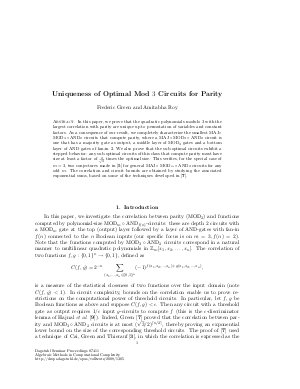Document# Uniqueness of Optimal Mod 3 Circuits for Parity

### Authors Frederic Green, Amitabha Roy## File

DagSemProc.07411.7.pdf
• Filesize: 213 kB
• 15 pages

## Cite As

Frederic Green and Amitabha Roy. Uniqueness of Optimal Mod 3 Circuits for Parity. In Algebraic Methods in Computational Complexity. Dagstuhl Seminar Proceedings, Volume 7411, pp. 1-15, Schloss Dagstuhl - Leibniz-Zentrum für Informatik (2008)
https://doi.org/10.4230/DagSemProc.07411.7

## Abstract

We prove that the quadratic polynomials modulo \$3\$ with the largest correlation with parity are unique up to permutation of variables and constant factors. As a consequence of our result, we completely characterize the smallest MAJ~\$circ mbox{MOD}_3 circ { m AND}_2\$ circuits that compute parity, where a MAJ~\$circ mbox{MOD}_3 circ { m AND}_2\$ circuit is one that has a majority gate as output, a middle layer of MOD\$_3\$ gates and a bottom layer of AND gates of fan-in \$2\$. We also prove that the sub-optimal circuits exhibit a stepped behavior: any sub-optimal circuits of this class that compute parity must have size at least a factor of \$frac{2}{sqrt{3}}\$ times the optimal size. This verifies, for the special case of \$m=3\$, two conjectures made by Due~{n}ez, Miller, Roy and Straubing (Journal of Number Theory, 2006) for general MAJ~\$circ mathrm{MOD}_m circ { m AND}_2\$ circuits for any odd \$m\$. The correlation and circuit bounds are obtained by studying the associated exponential sums, based on some of the techniques developed by Green (JCSS, 2004). We regard this as a step towards obtaining tighter bounds both for the \$m ot = 3\$ quadratic case as well as for higher degrees.
##### Keywords
• Circuit complexity
• correlations
• exponential sums

## Metrics

• Access Statistics
• Total Accesses (updated on a weekly basis)
0